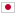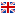Contest Duration: - (local time) (180 minutes) Back to Home
I - Obstruction/Time Limit: 2 sec / Memory Limit: 256 MB

### Problem

There is a two-dimensional grid of N \times N cells. Let (r, c) be the r-th row's c-th cell from the left. Some of these cells are painted black, other cells are painted white.

At first you are at (1, 1), and you want to go to (N, N). However, there's a stranger Mr.X trying to obstruct your way to (N, N).

You and Mr.X move in turns. At the beginning Mr.X starts the move. On each move each person can move as following.

• Mr.X moves to one of the white cell adjoining to the cell where you are. If there is no cell Mr.X can move to, Mr.X disappears from the grid for that turn.
• You can move to one of the cells adjoining to the cell where you are, which Mr.X didn't move to in his last turn.

In other words, you can move to one of the adjoining cell. But before your move Mr.X choose one of the possible move and block that. However, Mr.X can't block your move to a black cell.

You will be given the color of each cell. Determine if you can reach (N, N) no matter how Mr.X obstructs your way.

### Input

N
s_{(1,1)}s_{(1,2)}…s_{(1,N)}
s_{(2,1)}s_{(2,2)}…s_{(2,N)}
:
s_{(N,1)}s_{(N,2)}…s_{(1,N)}

• On the first line you will be given an integer N (2 \leq N \leq 1,000), the size of the grid.
• Following N lines shows the color of each cell. Each line consists of . and #. The i-th (1 \leq i \leq N) row's j-th (1 \leq j \leq N) character represents the color of (i, j). When the character is ., (i, j) is painted white. When the character is #, (i, j) is painted black.

### Output

If you can reach (N, N) no matter how Mr.X obstructs the way, output YES. If not, output NO. (Both without the period.)

Make sure to insert a line break at the end of the output.

### Input Example 1

4
..##
...#
#..#
####


### Output Example 1

YES


If Mr.X blocks (1, 2) at his first move, you can move to (2, 1). After that you can follow the black cells to reach (4, 4).

If Mr.X blocks (2, 1) you can move to (1, 2) and then follow the black cells to reach (4, 4).

Therefore, you can reach (4, 4) notwithstanding Mr.X's obstruction. The answer is YES.

### Input Example 2

4
..##
....
#..#
####


### Output Example 2

NO


In this case, when you are at (1, c), Mr.X can block (2, c). If Mr.X follows this optimal strategy for him, you can't reach (4, 4). The answer is NO.

### Input Example 3

2
.#
#.


### Output Example 3

NO


In this case, Mr.X can block (2,2).